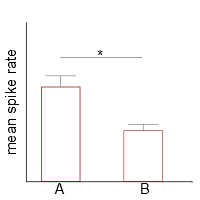Maybe it is because of the change in fields and scientific environment, but I increasingly doubt data presented in talks, posters and articles. Mostly because I find myself wondering whether the choice of statistical description of the data makes any sense. I am not speaking of advanced statistics at all, just the simplest descriptive techniques and the choice of the right plot for it.figure 1. X represents spike rates, categories cell types A and B respectively. Whisker indicates standard deviation.

My doubts are usually raised when I see a bar plot as sketched in figure 1. What looks like a rather okay representation of data actually does not really give you an intuitive idea of how the data are distributed.

A barplot is most usefull to represent counts in histograms or percentages. The bar is supposed to indicate that there would be data points spanning the whole range from the top of the bar down to the x-axis (figure 2). Standard deviations on a barplot would indicate that one measured the whole thing several times and there is some wiggle at the overall sum of each measurement. For example, if you counted the number of labeled neurons in the same brain area of several animals and you want to show the cell count numbers. Here, the relation of the sizes of the bars directly translate into the relation between count numbers.

However, the truth behind a plot might actually look more like I sketched in figure 3, especially when it comes to the comparison between the spike rates of two populations of neurons. A population of neurons in each category gathers around a certain value, while most of the value range is not representative of the data set. People actually are aware that this is a major flaw. Still, they keep making bar plots for traditional reasons. A somewhat half-baked attempt to rescue the presentation involves plotting the actual data into the bar plot, as I did in figure 3. This trend in bar plot presentation is what actually made me aware of how badly such plots describe the distribution of the data.

I suggest a pretty simple solution: use whisker plots (figure 4). Here, the mean value is indicated by a horizontal line and whiskers indicate the standard deviation above and below the mean. In contrast to the bar plot, there is no graphical element that envelopes a data range that is non-existent in your data set. Instead, the graphical elements envelope the data relative tightly and the difference between the distributions is more visible.

A more important issue is, when I find data that look like I sketched in figure 5. I have seen this in WAY too many occasions. Let us think about it: you pool the data from cell type A and describe it (obviously blindly) using the mean and standard deviation. Then you look at your data and… neither of these variable are represented in your data set.

Just to make clear what I mean: the mean and standard deviation are supposed to show where the main body of your data is to be found and how it is distributed, if they are no way near your data points, the description is plain wrong.

The dataset actually shows a bimodal distribution. One cluster is pretty much identical with the neurons from category B, the other one is significantly higher. I found exactly such comparisons very often, where one subpopulation looks like the other category while another is clearly different. But by bluntly pooling the data, the difference between categories is underestimated. Of course, if your six cells are now distributed over two categories, you need more data.

Either to see if you just missed cells that have intermediate firing or to have enough data to show better separation. But that’s how it is. There is no use in describing data you actually already have, in a bad way, just to get your ‘n’. It is better to say ‘this is how it looks now, we are increasing the statistical power of our set’ than to play ignorant. People. See. That.